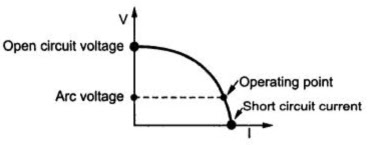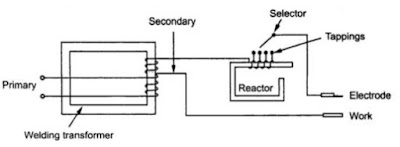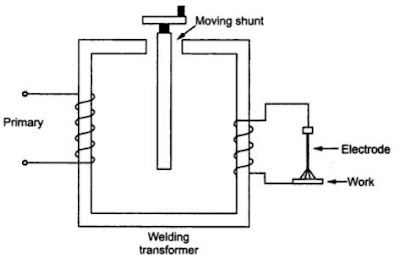# Welding Transformer Working Principle and Applications

## Welding Transformer Working Principle and Applications &characteristics of welding transformer

Now a days we have many ac power supplies. So the usage of welding transformer has significant role in welding compared to a motor generator set. When we need to use a motor generator set for welding we need to run it continuously which produces a lot of noise. With the help of welding transformer weld is done with a less noise. Now let us see in detail about welding transformer.

### Construction of welding transformer:

1. Welding transformer is a step down transformer.
2. It has a magnetic core with primary winding which is thin and has large number of turns on one arm.
3. A secondary winding with less number of turns and high cross-sectional area on the other arm.
4. Due to this type of windings in primary and secondary it behaves as step down transformer.
5. So we get less voltage and high current from the secondary winding output. This is the construction of ac welding transformer.
6.A dc welding transformer also has same type of winding the only difference is that we connect a rectifier(which converts ac to dc) at the secondary to get dc output.
7.We also connect a inductor or filter to smooth the dc current. This will be construction of dc welding transformer. The diagrams are shown below.Fig 1.DC welding transformerFig 2.AC welding transformer
Note:
Many people have a doubt which is primary winding and which is secondary winding. The winding which is connected to power supply is called primary winding and the winding to which load is connected is called secondary winding.

### Working of welding transformer:

1.As it is a step down transformer we have less voltage at secondary which is nearly 15 to 45 volts and has high current values which is nearly 200 A to 600 A it can also be higher than this value.
2. For adjusting the voltage on secondary side there are tappings on secondary winding by this we can get required amount of secondary current for welding.
3. These tappings are connected to several high current switches.
4. Now one end of secondary winding is connected to the welding electrode and the other end is connected to the welding pieces as shown in fig 2.
5.When a high current flows a large amount of  I2R heat is produced due to contact resistance between welding pieces and electrode.
6.Because of this high heat the tip of electrode melts and fills the gap between the welding pieces.
This is how a welding transformer works.

### Volt – ampere characteristics of welding transformer:

Figure given below shows the volt – ampere characteristics of welding transformer.### Arc control of welding transformer:

The impedance of welding transformer must be higher than the normal transformer to control arc and also to control current.
We can use different reactors for controlling the arc. They are
1.Tapped reactor.
2.Moving coil reactor.
3.Magnetic shunt reactor.
4. Continuously variable reactor.
5. Saturable Reactor.
Now let us see each of this methods for arc control of welding transformer in detail.

#### 1.Tapped reactor:

Below is the circuit for arc control using tapped reactor is given below.With the help of taps we control the current. It has limited current control.

#### 2. Moving coil reactor:

Below is the circuit for arc control using moving coil reactor.The distance between primary and secondary decides the amount of current. If the distance between the primary and secondary is high then the current is less.

#### 3. Magnetic shunt reactor:

Below is the circuit for arc control using magnetic shunt reactor.By adjusting the central magnetic shunt flux is changed. By changing the flux current can be changed.

#### 4. Continuously variable reactor:

Below is the circuit for arc control using continuously variable reactor.By varying the height of reactor core insertion is changed. If core insertion is greater reactance is higher so output current will be less.

#### 5. Saturable reactor:

Below is the circuit for arc control using saturable reactor.The reactance of the reactor in this is adjusted by changing the value of d.c. excitation which is obtained from d.c. controlled transducer. Higher the d.c. currents, reactor approaches to saturation. This changes the reactance of reactor. By changing the reactance current can be changed.
By using above reactors current can be controlled which helps to control the arc.
In this post we have learnt about welding transformers.